Thursday,  April 22

A quick review of

Surfaces:Mark Sudduth's web page of surfaces.[ A physics master's degree student at UT, Arlington.

Examples:A closed disc, an open disc, a plane, an annulus- cylinder, a mobius band;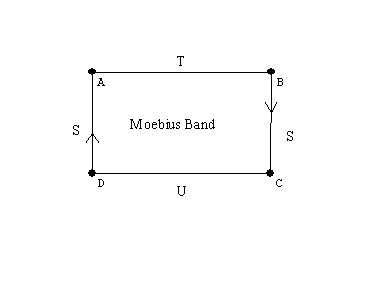a sphere,
a torus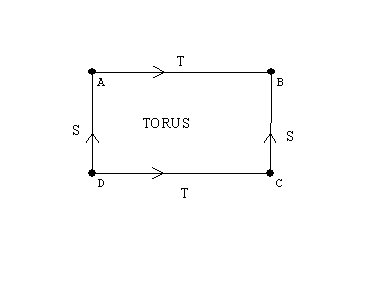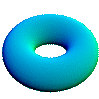a Klein bottle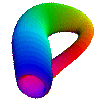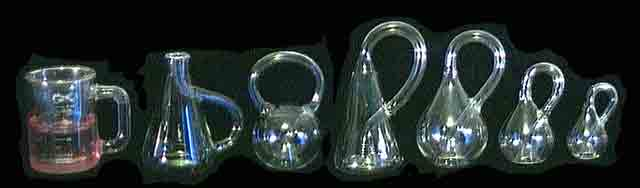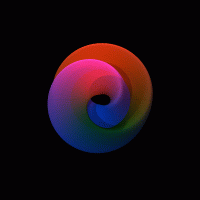,

the projective plane... Why is this a closed and bounded surface?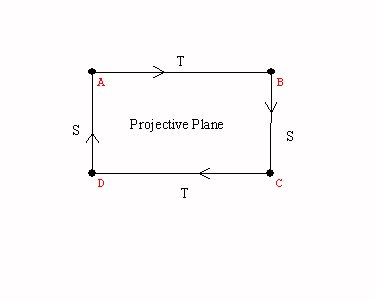A sphere with a cross cap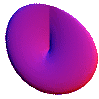.

Activity:Graphs on the torus.
Games and puzzles on the torus and the klein bottle.

Spheres with handles,
Making Klein Bottles activity.

Visualizations of surfaces by flattened - cut apart models.
A cylinder, a mobius band, the torus, the Klein bottle, the projective plane.

Closed Surfaces: Handles and cross-caps  attached to the sphere.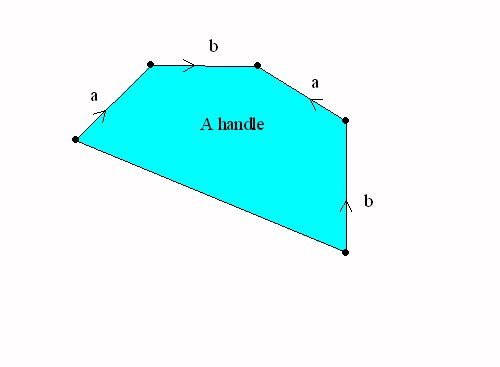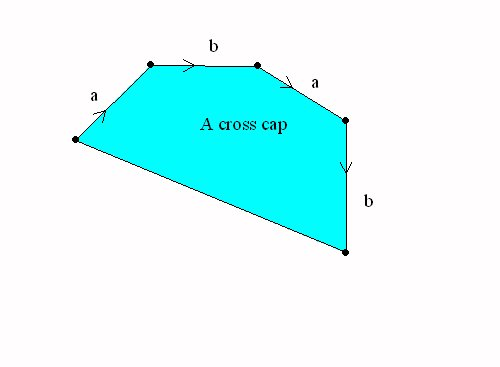A sphere with a handle = a torusA Sphere with a cross cap = the projective plane

The Topological Classification of "closed surfaces."

Every connected closed and bounded surface is topologically equivalent to a sphere with handles and crosscaps attached.

The Euler Characteristic of a surface:
This classification determines the Euler characteristic of each surface.

• If the surface is orientable, it is a sphere with n handles, so V-E+R = 1 - 2n +1 = 2-2n.  For example the torus has euler characteristic 2-2*1=0.

• If the surface is non-orientable, then  is it a sphere with k crosscaps and n handles, so the euler characteristic is V-E+R = 1 - (k+2n) +1 =2 -2n -k.
Notice that a sphere with two cross caps has euler characteristic 0, the same as the torus. But this was the Euler characteristic of the Klein Bottle.
So... we should be able to recognize the Klein bottle as a sphere with two cross caps.
This can be done by a single normalization of one pair of edges with the same orientation.

Other interest in surfaces: Examples
Ways to think of surfaces : cross-sections/ projections/moving curves/ using color to see another dimension.   ChromaDepthTM 3D

Generalization of surfaces are called "manifolds".  cross sections / projections/ moving surfaces-solids.
"minimal surfaces" (FAPP video)

Projective Geometry

Perspective from a point.... what happens to a circle in a plane?

The points on the circle and the center of perspective determine lines through the center.

The surface of these lines is a cone.

When you pass the cone through Flatland your see curves- the "conic sections".
Check out this applet.

These are....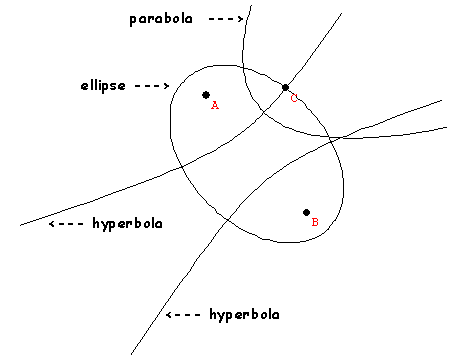Google "conics."   much is found!   :)

Show video on Conics  FAPP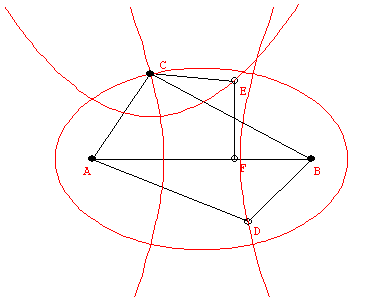The characterization of points on a conic by measurements are:
ellipse:  AC + BC = constant
parabola: EC = EF
hyperbola: |CB-CA| = constant.
Rope Conic Activity.
Using a rope :form a human ellipse.
After that demonstrate how to use a rope to form a hyperbola and then a parabola.

String drawing conic activity.